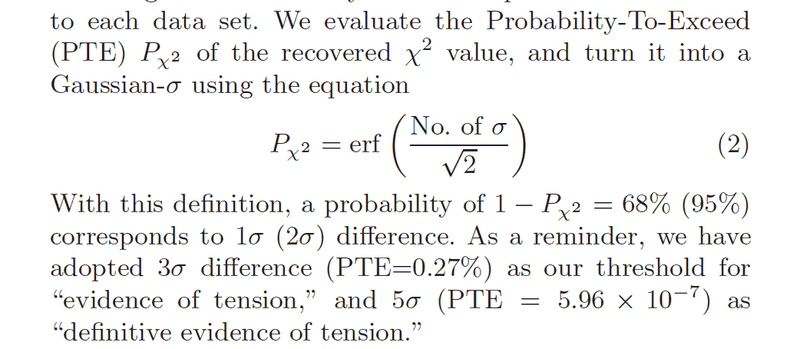# Probability-To-Exceed (PTE) and Chi^2 distribution

• I
I would like to know the difference between the ##\chi^{2}## distribution and the PTE (Probability-To-Exceed) ?For the moment, I only know the ##\chi^{2}## distribution with ##k=2## degrees of freedom :

and the relation with confidence level :

I don't know how to make the link with the image and text above. Indeed, in the article, they make appear the integral of gaussian whereas in ##(2)##, I can only make appear a simple integration of exponential (I mean, there is no "##\text{erf}##" function appearing unlike into the article).

If someone could explain the difference between ##\chi^{2}## distribution and ##P_{\chi^2}## PTE ?

Thanks

#### Attachments

Stephen Tashi
Even the additional information given on stackexchange https://stats.stackexchange.com/que...obability-to-exceed-pte-and-chi2-distribution doesn't clarify the situation. Questions about statistics involve what statistic is involved not merely what distribution is involved. To understand a statistic, one must know the data and how the statistic is computed from the data. In this example, it isn't clear what the data is. The stackexchange information seems to treat the "model parameters" as random variables. How does that work?

@Stephen Tashi

My main issue is to understand the method used in this article to be able to compare 2 data set and see if threre are :

1) there are Consistent since I have to extimate in both data sets the parameters contained into ##p_{A}## and ##p_{B}## vectors (parameters are shared into 2 experiments A and B)
2) there are Independant from a statistical point of view
3) Give consistent estimation of parameters with A and B experiments (roughly equal)

I would like to get more informations about the using of the Probability-To-Exceed ##Pr_{\chi^2}## in this topic. Up to now, I know classical relation between condifence level (CL) and the ##\chi^2 ## distribution with given number of degrees of freedom (with n.o.f = 2, the ##\chi^2## distribution has an exponential density function, and not gaussian like it seems to be the case here). So the relation I know is : ##1 - CL = Pr(d(\chi^2)) > d(\chi^2_CL))##. Must I consider the P-T-E as a similar principle of ##\chi^2## distribution but with a different density function (in this case here, density function of PTE is maybe gaussian) ?

By the way, if I take a large number of sigma in this PTE, then, I have a small value for PTE and then a high C.L (confidence level) : it is the expected result, right ? since the probability to exceed a value far away from the ##\chi^2_min## is small. Thanks for your explanations and help.

Anyone could understand my issue ? or maybe Is my question bad formulated.

I just want to get some advices about the notion of consistency or the general method to compare and do cross-correlations between 2 data set like it is performed in article cited above.
In the case of this article, I would like to understand f someone could explain the difference between ##\chi^{2}## distribution and ##P_{\chi^{2}}## Probability To Exceed (PTE) ?